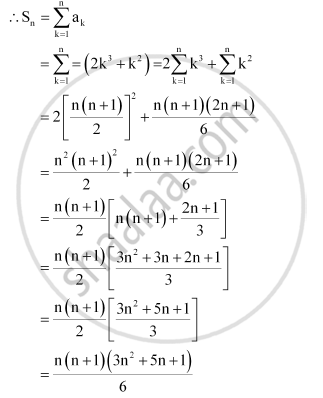CBSE (Arts) Class 11CBSE
Share
Notifications

View all notifications
Books Shortlist
Your shortlist is empty

# Find the Sum to N Terms of the Series 3 × 12 + 5 × 22 + 7 × 32 + … - CBSE (Arts) Class 11 - Mathematics

Login
Create free account

Forgot password?
ConceptSum to N Terms of Special Series

#### Question

Find the sum to n terms of the series 3 × 12 + 5 × 22 + 7 × 32 + …

#### Solution

The given series is 3 ×12 + 5 × 22 + 7 × 32 + …

nth term, an = ( 2n + 1) n2 = 2n3 + n2Is there an error in this question or solution?

#### APPEARS IN

NCERT Solution for Mathematics Textbook for Class 11 (2018 to Current)
Chapter 9: Sequences and Series
Q: 3 | Page no. 196
Solution Find the Sum to N Terms of the Series 3 × 12 + 5 × 22 + 7 × 32 + … Concept: Sum to N Terms of Special Series.
S